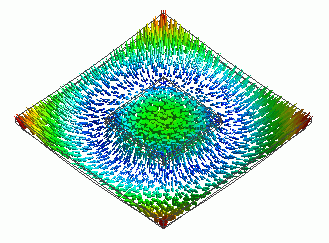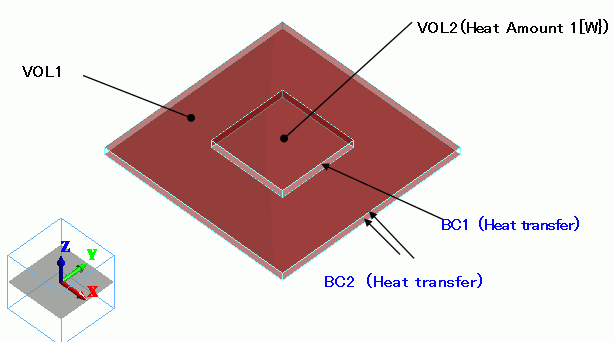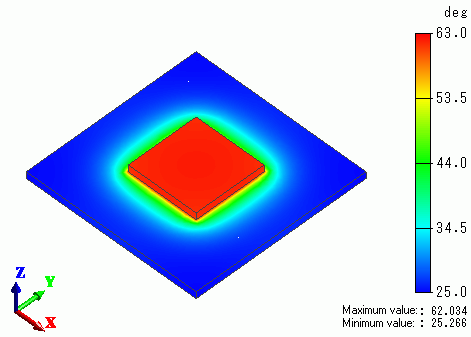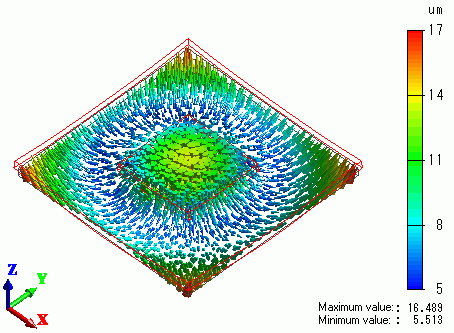﻿ Deformation due to the Temperature Gradient #2 – Multiple MaterialsExamples | Product | Murata Software Co., Ltd.# Example2Deformation due to the Temperature Gradient #2 – Multiple MaterialsGeneral

• A heating chip is mounted on a substrate. The temperature gradient caused by the heating chip is calculated by thermal analysis [Watt]. This is the same as Exercise 7 of thermal analysis.

• The temperature gradient caused by the heating chip is calculated by thermal analysis [Watt].
The result is forwarded to mechanical stress analysis [Galileo] as a thermal load.

• The deformation, the displacement and the mechanical stress are solved.

• Unless specified in the list below, the default conditions will be applied.

### Analysis Space

 Item Settings Analysis Space 3D Model unit mm

### Analysis Conditions

Select Thermal analysis and Mechanical stress analysis.

 Item Settings Solvers Thermal analysis [Watt] Mechanical stress analysis [Galileo] Thermal-Analysis Type Steady-State Analysis Options N/A *

* “Thermal Load” is selected by default for the thermal load-mechanical stress coupled analysis.

The Step/Thermal Load tab is set as follows.

 Tab Setting Item Settings Step/Thermal Load * Reference temperature 25[deg]

* The reached temperatures come from the thermal analysis.

### Model

The same as Exercise 7 of thermal analysis.### Body Attributes and Materials

 Body Number/Type Body Attribute Name Material Name 0/Solid VOL1 006_Glass_epoxy * 1/Solid VOL2 001_Alumina *

* Available from the Material DB

### Boundary Conditions

The heat transfer coefficients for the forced convection are calculated as follows. See [Heat Transfer/Ambient Radiation] for more information.

h = 3.86 x (V/L)0.5xC [W/m2/deg]

where

Air flow V=1[m/s]

On the top and bottom faces of VOL1: Characteristic length L=0.05, C=1 -> h=17.26
Top face of the heat source (VOL2): Characteristic length L=0.02, L’=0.015, C=1 * -> h=27.3

*

The thickness (d) of the speed boundary layer at the edges of the heat source is calculated as follows

d=0.0182x(L’/V)0.5= 2.3[mm]

This is close enough to the thickness of heat source, so we set C=1.

 Boundary Condition Name/Topology Tab Boundary Condition Type Settings BC1/Face Thermal Heat Transfer/Ambient Radiation Heat transfer coefficient: 17.26 [W/m2/deg] Ambient temperature: 25[deg] BC2/Face Thermal Heat Transfer/Ambient Radiation Heat transfer coefficient: 27.3 [W/m2/deg] Ambient temperature: 25[deg]

Thermal analysis is performed based on the boundary conditions below. The resulting temperature distribution is forwarded to mechanical stress analysis.

### Results

The temperature distribution as a result of Watt is shown below.The next figure shows the vectors of displacement as a result of Galileo following Watt.The temperature gradient causes the deformation.

﻿